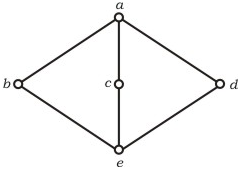Skip to content
Related Articles
GATE | GATE-CS-2005 | Question 90
• Last Updated : 11 Sep, 2014

The following is the Hasse diagram of the poset [{a, b, c, d, e}, ≤]The poset is

(A) not a lattice
(B) a lattice but not a distributive lattice
(C) a distributive lattice but not a Boolean algebra
(D) a Boolean algebra

Answer: (B)

Explanation:

```It is a lattice but not a distributive lattice.

Table for Join Operation of above Hesse diagram

V |a b c d e
________________
a |a a a a a
b |a b a a b
c |a a c a c
d |a a a d d
e |a b c d e

Table for Meet Operation of above Hesse diagram

^ |a b c d e
_______________
a |a b c d e
b |b b e e e
c |c e c e e
d |d e e d e
e |e e e e e

Therefore for any two element p, q in the lattice (A,<=)

p <= p V q ; p^q <= p

This satisfies for all element (a,b,c,d,e).

which has 'a' as unique least upper bound and 'e' as unique
greatest lower bound.

The given lattice doesn't obey distributive law, so it is
not distributive lattice,

Note that for b,c,d we have distributive law

b^(cVd) = (b^c) V (b^d). From the diagram / tables given above
we can verify as follows,

(i) L.H.S. = b ^ (c V d) = b ^ a = b

(ii) R.H.S. = (b^c) V (b^d) = e v e = e

b != e which contradict the distributive law.
Hence it is not distributive lattice.

so, option (B) is correct. ```

Attention reader! Don’t stop learning now. Learn all GATE CS concepts with Free Live Classes on our youtube channel.

My Personal Notes arrow_drop_up